Next: Unconstrained Optimization Up: Introduction Previous: Motivations   Contents

# Formal description

This thesis about optimization of non-linear continuous functions subject to box, linear and non-linear constraints. We want to find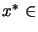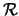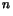which satisfies: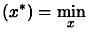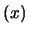Subject to: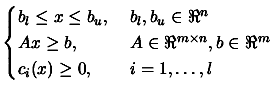(1.1)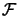is called the objective function.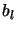andare the box-constraints.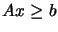are the linear constraints.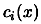are the non-linear constraints.
The following notation will be used: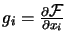is the gradient of.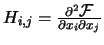is the Hessian matrix of.

The choice of the algorithm to solve an optimization problem mainly depends on:
• The dimension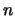of the search space.
• Whether or not the derivatives of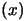are available.
• The time needed for one evaluation offor a given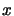.
• The necessity to find a global or a local minimum of.
• The noise on the evaluation of.
• Whether the Objective function is smooth or not.
• Whether the search space is continuous (there is no discrete variable like variable which can take the following values: red, green, blue.).
• The presence of (non-linear) constraints.
If there are lots of noise on, or if a global minima is needed, or if we have discrete variables we will use evolutionary algorithm (like genetic algorithms). These kind of algorithms can usually only have box constraints.

In the rest of the thesis, we will make the following assumptions:
• The objective function is smooth
• The non-linear constraints are cheap'' to evaluate
• We only want a local minimum.
An optimization (minimization) algorithm is nearly always based on this simple principle:
1. Build an approximation (also called local model'') of the objective function around the current point.
2. Find the minimum of this model and move the current point to this minimum. Go back to step 1.
Like most optimization algorithms, CONDOR uses, as local model, a polynomial of degree two. There are several ways of building this quadratic. CONDOR uses multivariate lagrange interpolation technique to build its model. This techniques is particularly well-suited when the dimension of the search space is low. When there is no noise on the objective function, we can use another, cheaper technique called BFGS update'' to construct the quadratic. It allows us to build local models at very low CPU cost (it's very fast).

We have made the assumption that an evaluation of the objective function is very expensive (in term of computing time). If this is not the case, we must construct a local model in a very short time. Indeed, it serves no point to construct a perfect local model using many computer resources to carefully choose the direction to explore. It's best to use an approximate, cheap to obtain, search direction. This will lead to a little more function evaluations but, since they are cheap, the overall process will be faster. An example of such algorithm is the ''Rosenbrock's method''. If the objective function is very cheap to evaluate, it's a good choice. You will find in the annexe (section 13.9) a personal implementation in C++ of this method. Algorithms based on the BFGS update'' are also able to construct a good model in a very short time. This time can still become not negligible when the dimension of the search space is high (greater than 1000). For higher dimension, the choice of the algorithm is not clear but, if an approximation of the Hessian matrixof the objective function is directly available, a good choice will be a Conjugate-gradient/Lanczos method.

Currently, most of the researches in optimization algorithms are oriented to huge dimensional search-space. In these algorithms, we construct approximate search direction. CONDOR is one of the very few algorithm which adopts the opposite point of view. CONDOR build the most precise local model of the objective function and tries to reduce at all cost the number of function evaluations.

One of the goals of this thesis is to give a detailed explanation of the CONDOR algorithm. The thesis is structured as follows:
• Unconstrained Optimization: We will describe the algorithm in the case when there are no constraints. The parallelization of the algorithm will also be explained.
• Chapter 2: A basic description of the CONDOR algorithm.
• Chapter 3: How to construct the local model of the objective function? How to assess its validity?
• Chapter 4: How to compute the minimum of the local model?
• Chapter 5: When we want to check the validity (the precision) of our local model, we need to solve approximatelysubject to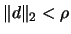. How do we do?
• Chapter 6: The precise description of the CONDOR algorithm.
• Chapter 7: Numerical results of the CONDOR algorithm on unconstrained problems.
• Constrained Optimization:
• Chapter 8: We will make a short review of algorithms available for constrained optimization and motivate the choice of our algorithm.
• Chapter 9: Detailed discussion about the chosen and implemented algorithm.
• Chapter 10: Numerical Results for constrained problems.
• The METHOD project (chapter 11)
The goal of this project is to optimize the shape of the blades inside a Centrifugal Compressor (see illustration of the compressor's blades in Figure 11.1). This is a concrete, industrial example of use of CONDOR.
• Conclusion (chapter 12)
• Annexes (chapter 13)
• Code (chapter 14)Next: Unconstrained Optimization Up: Introduction Previous: Motivations   Contents
Frank Vanden Berghen 2004-04-19